# Registration method for three-dimensional point cloud in rough and fine registrations based on principal component analysis and iterative closest point algorithm

Registration method for three-dimensional point cloud in rough and fine registrations based on principal component analysis and iterative closest point algorithm

Heqiang Tian Xiaoqing Dang Jihu Wang Dongmei Wu

College of Mechanical and Electronic Engineering, Shandong University of Science and Technology, Qingdao 266590, China

Qingdao Municipal Hospital Group, Qingdao 266073, China

State Key Laboratory of Robotics and System, Harbin Institute of Technology, Harbin 150080, China

Page:
57-75
|
DOI:
https://doi.org/10.3166/TS.34.57-75
|
Accepted:
|
Published:
30 June 2017
| Citation

OPEN ACCESS

Abstract:

For the registration between the preoperative computed tomography (CT) image and the intraoperative patient space in surgical navigation technology, this paper puts forward a registration technique based on the principal component analysis (PCA) and the iterative closest point (ICP) algorithm, using two feature point clouds from the medical image space and the actual patient space. Firstly, the feature point cloud of the image space was obtained through the reconstruction, segmentation and interactive operation of the CT image, while the corresponding feature point cloud in the actual patient space was collected by the optical locator in real time. Secondly, the eigenvectors of the two sets of point clouds were obtained through the PCA for rough registration, and the optimal solution of the registration matrix was found by the ICP. Finally, the effect of the proposed registration method was verified against point cloud data (the surgical navigation accuracy was evaluated through an experiment on the vertebra model), and the impacts of the number of point clouds and Gaussian noise on the registration error were investigated in details. The results show that the proposed method is simple and capable of realizing high registration accuracy, and completed registration with a less-than-2mm error in our experiment; in addition, the registration accuracy was greatly affected by the number of point clouds and the noise of the photoelectric locator. This research provides a general solution for registration in surgical navigation and lays the theoretical basis for improving intraoperative registration accuracy.

Keywords:

intraoperative registration, principal component analysis (PCA), iterative closest point (ICP) algorithm, point cloud, gaussian noise.

1. Introduction

Surgical navigation, an integration of stereotactic technology, modern imaging technology and computer technology, assists doctors in performing well-planned minimally invasive surgeries. The success of surgical navigation hinges on the intraoperative registration between images, patients and devices, especially the spatial transformation between images and patients. In other words, the registration technique and accuracy between the space of computed tomography (CT) images and that of patients, i.e. the one-to-one correspondence between positions in CT model space and those in actual space, determines the robustness, feasibility and reliability of the entire surgical navigation system (Goerlach et al., 2016).

The existing intraoperative registration methods are either marker-based registration or marker-free registration (Luebbers et al., 2008; Zheng et al., 2007). The marker-based registration (Marmulla et al., 2005; Schramm et al., 1999) calculates the transformation parameters using the coordinates of some special additional markers (e.g. metal balls). This method is easy to implement and conducive to automatic and efficient registration. The marker-free registration can be further divided into landmark matching and surface matching.

The landmark matching method relies on geometric features (e.g. points, lines and faces) in the same part of the human body or the defined registration area. During the surgery, the spatial positions of the same geometric features on the patient are measured by the positioning device, and the transformational relationship is determined between the image coordinate system and spatial coordinate system of these features. Clinical surgeons often implant markers that can be recognized by an image scanning device on the patient’s spine or directly use anatomical landmarks. At least 4 pairs of points should be selected respectively from the patient’s spine and the 3D image reconstructed by computer, such that the computer could find the correspondence between the two sets of coordinates by a specific algorithm. In general, the landmark matching method features simple implementation, accurate solution, precise registration (which is positively correlated with the pairs of points) and high stability. Nevertheless, this method sometimes fails to determine the exact correspondence via point registration when the anatomical landmarks are not clear, the additional marker coordinates are not accurately detected, or the artificial markers are difficult to add.

The surface matching method is an extension of the iterative closest point (ICP) algorithm proposed by Besl and McKay (1992). By this method, the image coordinates of the feature surface are derived from the image, and then the spatial coordinates of the surface points are obtained by the positioning device; next, the optimal transformational relationship between the image coordinates and image coordinates is determined through the iterative minimization of the distance between the two sets of coordinates. Unlike point registration, the ICP does not require the exact correspondence between two point sets. Instead, the iteration starts on an initial correspondence and uses the registration between corresponding point sets: first, determine the set of the closest points corresponding to the set of measured points; then, determine the set of the closest points corresponding to that set by optimal analysis; finally, find an optimized correspondence and an optimized registration result.

Despite the good registration effect on the point sets whose exact correspondence is difficult or impossible to obtain, the ICP is low in computing efficiency and prone to local minimum. So far, many scholars have improved the ICP algorithm and achieved certain results. To reduce the impact of mismatched points, Marani et al., (2016) assumed that the distance error of the matching points obeys the zero-mean normal distribution and uses the 3σ criterion to remove the mismatched points. Mohammadzade et al., (2013) viewed the points in the pair with the smallest Euclidean distance as the potential matching points, adopted the neighborhood points with the same normal vector as the matching points, and obtained the rigid body transformation matrix via singular value decomposition. Considering the sensitivity of the ICP to the initial iterative values, FitzGibbon (2001) replaced singular value decomposition with Levenberg Marquardt (LM) algorithm to optimize nonlinear error function, which significantly improves global convergence. To reduce the ICP’s computational cost, Jost & Heinz (2008) put forward a neighborhood search algorithm based on time complexity, realized the fast search for matching points, and improved the real-time performance of the ICP. Rusinkiewicz & Levoy, (2001) proposed a fast registration method based on point-to-projection search for the nearest neighbors, which greatly improves the registration efficiency. Li & Gfiffiths, (2000) presented an iterative closest line (ICL) algorithm, in which the registration is realized through directly connecting points in two point clouds and finding corresponding line segments.

To mitigate the sensitivity of the ICP to the initial iterative values, this paper introduces rough registration before accurate registration. Specifically, the initial conditions were realized through principal component analysis (PCA) based on the feature points. After the rough registration, the accurate surface matching was conducted through iterative point method, aiming to minimize the rotation misalignment between the CT image space and the patient space and overcome the local convergence of the ICP. After introducing the principle of the registration algorithm in PCA-ICP rough and fine registrations, the author provided the registration method based on feature point cloud, and then verified the proposed intraoperative registration method by vertebra model. The registration results were subjected to an analysis of influencing factors.

2. Materials and method

2.1 Principle of ICP registration algorithm

2.1.1. Corresponding point set registration algorithm

The registration of corresponding point sets can be abstracted into the following mathematical problem. Let W={wi} and M={mi}(i=1,2,…n) be the coordinates of a point set in the patient coordinate system and the image coordinate system, respectively, where n is the number of registration points. The problem is to find the optimal transformation matrix TW-M such that the transformed coordinates TW-M(wi) are as close as possible to coordinates of mi. After determining the correspondence between the target point cloud and the reference point cloud, the optimal registration parameters between the two point sets should be determined by a proper optimization method. For the said mathematical problem, the commonly used optimization methods include the unit quaternion method introduced by Berthold and the singular value decomposition (SVD) method.

Inspired by the unit quaternion theory, this paper relies on the least squares method to derive the optimal transformation matrix between the two coordinate systems. In the quaternion method, four rotation parameters are used to represent the rotation transformation and three translation parameters to represent the translation transformation in the rigid body transformation matrix TW-M. All these parameters are placed in a vector q=[qR, qT], where qR is the rotation matrix represented by the quaternion and qT is the translation vector represented by the three translation parameters. Then, the mathematical problem can be transformed into the search for the minimum value of the objective function f(q):

$f ( q ) = \frac { 1 } { n } \sum _ { i = 1 } ^ { n } \left\| m _ { i } - \left( q _ { R } w _ { i } + q _ { T } \right) \right\| ^ { 2 }$     (1)

The mathematical problem is solved in the following steps:

(1) Calculate the centroid w0 of point set W and that m0 of point set M:

$w _ { 0 } = \frac { 1 } { n } \sum _ { i = 1 } ^ { n } w _ { i } \quad m _ { 0 } = \frac { 1 } { n } \sum _ { i = 1 } ^ { n } m _ { i }$    $i = 1,2 , \cdots , n$        (2)

(2) Calculate the covariance matrix C of the two point sets W and M:

$C = \sum _ { i = 1 } ^ { n } \left[ \left( m _ { i } - m _ { 0 } \right) \left( w _ { i } - w _ { 0 } \right) ^ { \mathrm { T } } \right] = \sum _ { i = 1 } ^ { n } m _ { i } w _ { i } ^ { \mathrm { T } } - m _ { 0 } w _ { 0 } ^ { \mathrm { T } } = \left[ \begin{array} { c c c } { c _ { 11 } } & { c _ { 12 } } & { c _ { 13 } } \\ { c _ { 21 } } & { c _ { 22 } } & { c _ { 23 } } \\ { c _ { 31 } } & { c _ { 32 } } & { c _ { 33 } } \end{array} \right]$      (3)

(3) Construct the symmetric matrix E from the covariance matrix C:

$E = \left[ \begin{array} { c c c c } { \operatorname { tr } ( C ) } & { c _ { 23 } - c _ { 32 } } & { c _ { 31 } - c _ { 13 } } & { c _ { 12 } - c _ { 21 } } \\ { c _ { 23 } - c _ { 32 } } & { 2 c _ { 11 } - \operatorname { tr } ( C ) } & { c _ { 12 } + c _ { 21 } } & { c _ { 13 } + c _ { 31 } } \\ { c _ { 31 } - c _ { 13 } } & { c _ { 12 } + c _ { 21 } } & { 2 c _ { 22 } - \operatorname { tr } ( C ) } & { c _ { 23 } + c _ { 32 } } \\ { c _ { 12 } - c _ { 21 } } & { c _ { 13 } + c _ { 31 } } & { c _ { 23 } + c _ { 32 } } & { 2 c _ { 33 } - \operatorname { tr } ( C ) } \end{array} \right]$       (4)

Where tr(C) is the trace of the covariance matrix C, i.e. the sum of the diagonal elements of that matrix.

(4) Calculate the eigenvalue and eigenvector of the symmetric matrix E:

According to Berthold’s algorithm, the rotation matrix qR represented by the quaternion Q(q0, qx, qy, qz) minimizes the value of the objective function f(q), when the quaternion equals the eigenvector corresponding to the maximum eigenvalue of the symmetric matrix E. The quaternion-based solution to matrix qR can be expressed as:

$q _ { R } = \left[ \begin{array} { c c c } { q _ { 0 } ^ { 2 } + q _ { x } ^ { 2 } - q _ { y } ^ { 2 } - q _ { z } ^ { 2 } } & { 2 \left( q _ { x } q _ { y } - q _ { 0 } q _ { z } \right) } & { 2 \left( q _ { x } q _ { z } + q _ { 0 } q _ { y } \right) } \\ { 2 \left( q _ { x } q _ { y } + q _ { 0 } q _ { z } \right) } & { q _ { 0 } ^ { 2 } + q _ { y } ^ { 2 } - q _ { x } ^ { 2 } - q _ { z } ^ { 2 } } & { 2 \left( q _ { y } q _ { z } + q _ { 0 } q _ { x } \right) } \\ { 2 \left( q _ { x } q _ { z } - q _ { 0 } q _ { y } \right) } & { 2 \left( q _ { y } q _ { z } + q _ { 0 } q _ { x } \right) } & { q _ { 0 } ^ { 2 } + q _ { z } ^ { 2 } - q _ { x } ^ { 2 } - q _ { y } ^ { 2 } } \end{array} \right]$          (5)

According to the centroid w0 of point set W and that m0 of point set M, the translation matrix qT can be derived from qR:

$q _ { T } = m _ { 0 } - q _ { R } w _ { 0 }$            (6)

(5) Calculate the registration error:

After obtaining the values of the rotation matrix qR and the translation matrix qT according to the following formula:

$E _ { W - M } = \frac { 1 } { n } \sum _ { i = 1 } ^ { n } \left| m _ { i } - \left( q _ { R } w _ { i } + q _ { T } \right) \right|$     (7)

2.1.2. ICP registration algorithm

The ICP algorithm is an advanced registration method based on free-form surface. It has a good effect on the registration of the point sets whose exact correspondence is difficult or impossible to obtain. For the registration between the CT image space and the patient space, the point set of the patient space and that of the image space were defined as W={wi, i=0,1,2,…n} and M={mj, j=0,1,2,…k}, respectively, with n and k being the number of registration points. There is not necessarily a one-to-one correspondence between the elements of W and those of M. In addition, it is assumed that kn because the number of elements in the two sets does not have to be the same. The registration is to obtain the rotation and translation transformation matrices of the two spaces. The ICP registration algorithm can be described as follows:

(1) Read the two point sets to be registered:

First, obtain a set of coordinate points W={wi, i=0,1,2,…n} from the patient coordinate system and a set of coordinate points M={mj, j=0,1,2,…k} from the image coordinate system; then, read the two point sets to be registered and treat them as the input data for ICP algorithm.

(2) Calculate the closest points:

Search for the closest point in the set M to each point in the set W by Delaunay triangulation, and collect all these points in the set M into a new set of coordinate points M’={mr, r=0,1,2,…n}.

(3) Calculate the transformation matrices:

After determining the correspondence between the points, calculate the transformation relationship between the sets of coordinate points W and M’ by the unit quaternion optimization method such that

$\min \sum _ { s = 1 } ^ { n } \left\| m _ { s } ^ { \prime } - \left( R \left( w _ { s } \right) + T \right) \right\| ^ { 2 }$

In the resulting two transformation matrices, R is a 3´3 rotation matrix, and T is a 3´1 translation matrix.

(4) Update the set of coordinate points:

Transform the coordinates of the set of coordinate points W by the transformation matrices R and T, forming the new set of coordinate points W’={wt, t=0,1,2,…n}, where wt=R(wt)+T.

(5) Calculate the root mean square error:

Calculate the root mean square error between W’ and M’ and terminate the iteration if the error is smaller than the pre-set limit e.

2.1.3. PCA-ICP registration algorithm

The optimal registration matrix was searched for through coarse registration and fine registration.

(1) Coarse registration

Considering the strict requirements of the iterative algorithm on the initial value, the coarse registration provides an iterative initial value near the global optimal solution, with the aim to prevent the local minimum and reduce the computing time. Here, the two sets of coordinate points W and M are regarded as the sample values of two 3D random vectors. Next, three eigenvectors were obtained for the two sets by the PCA (Huizinga et al., 2016), and four corresponding pairs of feature points were identified according to the respective centers of gravity. After that, the initial registration matrix T0 was derived through the previously mentioned registration method of the corresponding point set.

(2) Fine registration

The number of iterations, the number of elements in W, the number of elements in M and the pre-set accuracy are denoted as k, Nw, NM and e, respectively.

① Let k=0, M0=M and T0=T0. Find the points with the closest Euclidean distance to points mi0 (i=1,2,…,NM) in M0 using the K.D. tree (Rajendra et al., 2014), forming a point set:

$D ^ { 0 } ( i ) = w _ { j }$             (8)

$\min _ { 1 \leq j \leq N _ { W } } \left\| m _ { i } ^ { 0 } - w _ { j } \right\|$            (9)

Set the initial residual:

$r ^ { 0 } = \frac { 1 } { N _ { M } } \sum _ { i = 1 } ^ { N _ { M } } \left\| m _ { i } ^ { 0 } - D ^ { 0 } ( i ) \right\|$          (10)

② Repeat the above steps to compute the situation under k+1:

$M ^ { k + 1 } = T ^ { k } \cdot M ^ { k }$     (11)

$D ^ { k + 1 } ( i ) = w _ { j }$    (12)

$\min _ { 1 \leq j \leq N _ { W } } \left\| m _ { i } ^ { k + 1 } - w _ { j } \right\|$        (13)

Then, the mean residual can be obtained as:

$r ^ { k + 1 } = \frac { 1 } { N _ { M } } \sum _ { i = 1 } ^ { N _ { M } } \left\| m _ { i } ^ { k + 1 } - D ^ { k + 1 } ( i ) \right\|$           (14)

③ Terminate the algorithm if |rk+1-rke or k reaches the upper bound of the number of iterations. Then, Tk·Tk-1·…T0 is the final registration matrix. Otherwise, go to step ④.

④ Find the registration matrix T in homogeneous coordinates by applying the quaternion method on the point sets corresponding to Mk+1 and Dk+1. Let Tk+1=T and go to step ②.

2.2 Registration method based on feature point cloud

For surgical navigation, the registration can be realized by the above algorithm after extracting the feature elements of the image space and the patient space.

2.2.1. Establishment of registration coordinate system

Registration is the basic problem in the implementation of surgical navigation. The core task is to find the relationship between the independent coordinate systems of different devices, unify them in a coordinate system, and realize the spatial transformation between them.

## 1.jpgFigure 1. Registration coordinate system for surgical navigation

The established registration coordinate system for surgical navigation is shown in Figure 1. Specifically, a model coordinate system {W} was set up in the computer image space, a patient coordinate system {P} was built in the surgical part of the patient, and an optical measurement coordinate system {M}was created on the optical positioner. The patient coordinate system {P} was determined by a reference rigid body. The rigid body is fixed and passive, and has its own coordinate system {S}. In addition, the robot end effector installed with the passive rigid body was defined as a tracking tool coordinate system {T}. The entire system takes the optical measurement coordinate system {M} as the absolute reference system, and relies on optical positioning technology to achieve spatial registration between the above coordinate systems.

2.2.2. Acquisition of feature surface point cloud from the image space

The preoperative CT image exists in the format of DICOM (Digital Imaging and Communications in Medicine). With self-defined geometric coordinates, the image is a 3D discrete gray field obtained through discrete sampling of continuous space. The 3D model of the spine was reconstructed using the Marching Cubes algorithm (Newman & Hong, 2006). This model is a surface rendering model, whose geometric description is based on the coordinate system defined before the scanning of CT data. After that, an interactive mouse point acquisition method was developed using the VTK visualization toolkit, in an attempt to obtain the coordinates of the 3D point set in the feature area for model reconstruction. By this method, the 3D point cloud W on the feature area of the reconstructed model can be picked up interactively, completing the digital acquisition from the feature surface of the image space.

2.2.3. Acquisition of feature surface point cloud from the patient space

The feature surface point cloud from the patient space was acquired by the optical locator Polaris Spectra (rated positioning accuracy<0.25mm) developed by the Canadian company NDI. As a passive infrared optical stereo positioning system, Passive Polaris describes the attitude transformation parameters of coordinate transformation with the 3D coordinates and Euler angles of spatial points, as well as the 3D coordinates and quaternion information. Under optical tracking, the surgical entity and the probing tool were linked together to form a rigid body. Therefore, the posture of the surgical entity against the probing tool can be obtained by the spatial variation technique of optical tracking. Specifically, the probe was swiped on a pre-set feature surface (corresponding to the feature surface on the reconstructed model) in the surgical part of the patient, such that the locator could record in real time the point cloud M in the actual workspace, completing the digital acquisition from the feature surface of the patient space. To obtain quality point cloud data, a certain threshold was set for the sampling distance threshold so that the point cloud obeys a relatively uniform distribution. Note that, in actual surgery, the area characterized by M only needs to be a subset of that characterized by W, owing to the limitations of the surgical operation area and the specific situation of intraoperative exposure.

2.2.4. Registration process

As shown in Figure 2, the feature areas are not necessarily continuous after the feature elements were extracted from the two spaces. Using the proposed algorithm, the obvious anatomical feature points on the spine surface were adopted first for rough registration, producing the initial transformation matrix. On this basis, the registration accuracy was enhanced by the ICP to derive the registration matrices for W and M. Then, it is possible to transform the coordinates in the virtual image space of the computer with those in the surgical site, that is, achieving the registration purpose. The ICP ensures the convergence to the target value, for the distance between the corresponding points is reduced in each iteration. Moreover, the correspondence is improved through each search of the nearest point. The registration process for surgical navigation is shown in Figure 3 below.

## 2a.jpg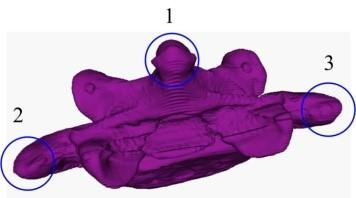(a) 3 feature areas of the spine model

## 2b.png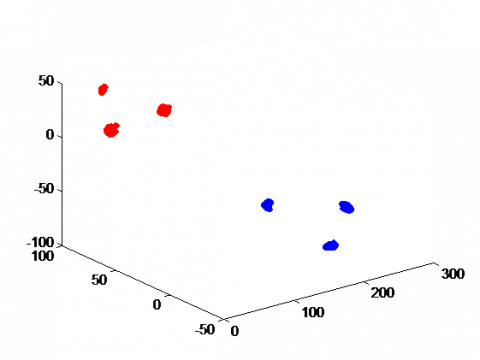(b) Point cloud of each feature area

Figure 2. Point clouds in feature areas

## 3.jpg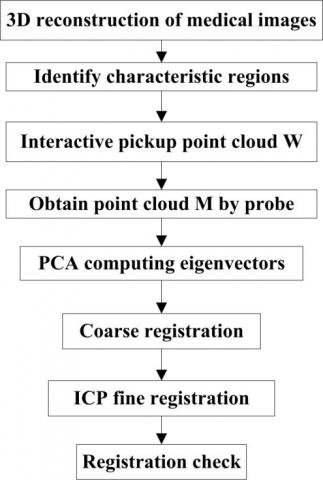Figure 3. Registration process for surgical navigation

3. Verification and results analysis

3.1 Verification of PCA-ICP registration algorithm

The ICP algorithm has a strict requirement on the initial value. If the rotation and translation misalignments between the two point sets to be matched are too large, the accuracy and convergence speed of the algorithm will be inevitably influenced, adding to the probability of falling into the local optimum trap. To verify the effect of the PCA-ICP registration algorithm, a set of a given number of points was adopted and denoted as the reference point set (blue point cloud). Then, the reference point set underwent rotation and translation transformations, forming the target point set (red point cloud). In addition to the translation transformation, the target point set was rotated by 60° around the Z axis with respect to the reference point set. After that, the ICP algorithm and the PCA-ICP algorithm were adopted to register the reference point set to the target point set. The registration effects of the two algorithms are shown in Figures 4 and 5. It can be seen that the PCA-ICP algorithm outperformed the ICP algorithm in the registration effect when the two point sets had a large rotational misalignment. Only the PCA-ICP algorithm managed to eliminate this misalignment.

## 4a.jpg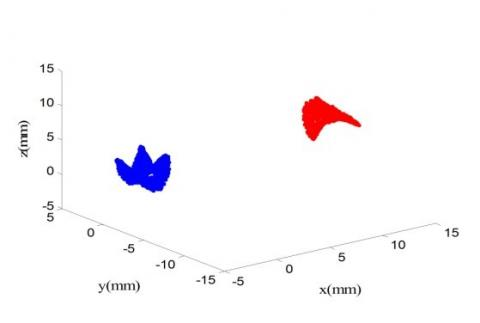(a) Pre-registration

## 4b.jpg(b) Post-registration

Figure 4. Registration effect of the ICP algorithm

## 5a.jpg(a) Pre-registration

## 5b.jpg(b) Post-registration

Figure 5. Registration effect of the PCA-ICP algorithm

3.2. PCA-ICP registration based on feature point cloud

The PCA-ICP algorithm based on feature point cloud was adopted for the registration experiment on a vertebra model. Firstly, the spine model was subjected to the CT scan at the layer spacing of 0.625mm and the pixel pitch of 0.439mm. Then, the 3D surface model of the spine model was established by the proposed interactive 3D reconstruction method. After that, three obvious features of the reconstruction model were selected as registration areas for the registration experiment (Figure 6). The interactive mouse point acquisition method was employed to collect the registration point cloud W (1,238 points) from the three feature areas as shown in Figure 6. The collected sample serves as the registration point set of the image space. Meanwhile, the point cloud M (1,377 points) was sampled by a probe in the patient space, which serves as the registration point set of that space.

## 6.png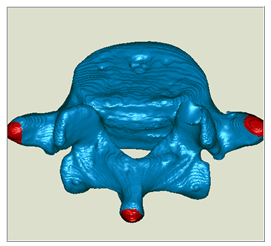Figure 6. Three feature areas

Figure 7(a) shows the distribution of registration point sets of the image space and the patient space before the registration, while Figure 7(b) displays the registration effect of the ICP algorithm on the two spaces. It can be seen that the point set of the image space overlapped that of the patient space, indicating that the PCA-ICP algorithm is a feasible way to register between the two spaces in an efficient and accurate manner.

## 7a.jpg(a) Pre-registration

## 7b.jpg(b) Post-registration

Figure 7. Registration results between image space and patient space

3.3 Verification of the registration accuracy of the PCA-ICP algorithm

Since metal balls can be easily recorded on CT images, five metal balls were pasted on the spine model to verify the registration accuracy. The 3D model of the spine model was generated through 3D reconstruction of the image (Figure 8). Then, the coordinates of the steel balls were calculated in the image space and the patient space. To eliminate the impact of random errors, ten groups of coordinate data were obtained for each steel ball, and the mean value was taken as the coordinates (Table 1). Let vPi be the coordinates of the i-th steel ball in the image space, TPi be the coordinates of the i-th steel ball after the rough and fine registrations by the ICP based on the feature point clouds, and dist(vPi, TPi) be the distance between these two types of coordinates (i=1,2,…5). Then, the mean registration error can be defined as:

$E r = \frac { 1 } { 5 } \sum _ { 1 } ^ { 5 } \left( \operatorname { dist } \left( ^ { v } P _ { i } , ^ { T } P _ { i } \right) \right)$     (15)

## 8a.jpg(a) Spine model

## 8b.jpg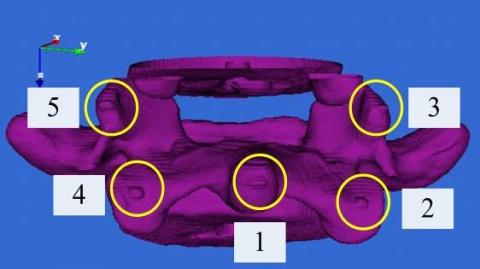(b) Reconstructed model

Figure 8. Spine model with steel balls and its 3D reconstructed model

Table 1. Registration errors for spine model

 No Δx (mm) Δy (mm) Δz (mm) ΔL (mm) 1 1.21 1.3 0.8 1.52 2 1.33 0.6 1.25 1.92 3 -0.8 -1.3 0.9 1.77 4 0.75 -0.82 1.4 1.78 5 1.1 0.7 -1.2 1.77

Ten ICP registration experiments were performed on the vertebra, and the mean registration error of each steel ball was calculated by equation (15). As shown in Table 1, the maximum average error was 1.75mm. The result falls in the allowable error range specified in previous references: Herring et al., (1998) pointed out that the ICP-based surface matching can achieve a less-than-2mm registration error, due to the large curvature change on the skeletal surface of the human body; the ICP registration error usually falls below 2mm, as stated in References (Tamura et al., 2005; Sugano et al., 2010). Through comparison, it is learned that the proposed algorithm can reliably and accurately complete the registration transformation between the image space and the patient space.

3.4 Analysis of factors affecting registration error

3.4.1. Impact of the number of registration points on registration error

Four obvious anatomical points were selected by a probe from the spine surface. The coordinates of these points were obtained in the virtual space and the actual space, and the initial matrix was calculated based on these data. After that, a series of points were taken from the spine surface for ICP registration against the initial matrix. During the ICP registration, the collected points were distributed evenly on a vertebra. 5 points firstly were collected from the vertebra, and next 5 points were added each time as a registration point until 100 points are reached finally. The registration points were subjected to ICP registration separately and the registration errors were computed. Let vPi be the coordinates of the i-th steel ball in the virtual space, TPi be the coordinates of the i-th steel ball after the ICP registration, and dist(vPi, TPi) be the distance between these two types of coordinates. Then, the mean registration error can be defined as:

$R M S = \frac { 1 } { n } \sum _ { 1 } ^ { n } \left( \operatorname { dist } \left( \stackrel { v } { P _ { i } } , ^ { T } P _ { i } \right) \right)$    (16)

## 9.jpg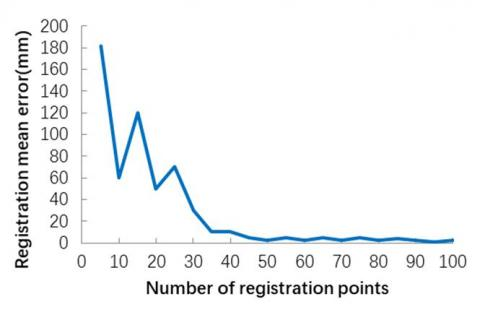Figure 9. Registration error curve of the spine model

The registration error curve in Figure 9 shows that: the registration error exhibited a declining trend with the increase in the number of registration points; the registration effect was dampened if the target point set fails to cover many of the points in the source set; the initial error is positively correlated with the number of points needed for registration. In light of these, the target point set should be located in the actual sampling area as much as possible for actual registration, and the initial registration matrix should be as accurate as possible.

3.4.2. Impact of random noise on registration error

The point cloud M collected by binocular stereo vision method tends to have many noises and outliers. To evaluate the impact of the optical locator noise on the registration matrix, the positioning error of the optical locator was simulated by adding zero-mean Gaussian noise to the point cloud M before the registration. Let T and T+ΔT be the registration matrices obtained before and after the addition of noise, respectively, and W and M be the point sets from the image space and the patient space, respectively. Then, the impact of the added noise on the registration error can be calculated according to equation (17). The registration errors at different levels of Gaussian noise are illustrated in Figure 10. It can be seen that the registration error increased with the standard deviation of Gaussian noise, reached the maximum when the latter surpassed 0.5 and remained stable thereafter.

$E ( \Delta T ) = \| W - ( T + \Delta T ) \bullet W \| = \| \Delta T \bullet W \|$      (17)

## 10.jpg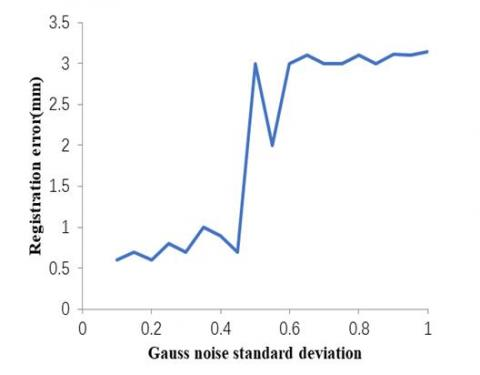Figure 10. Registration errors at different levels of Gaussian noise

3.4.3. Analysis of error factors

The above analysis reveals that the model registration experiment is affected by the following factors.

(1) Image scanning accuracy:

The 3D reconstruction accuracy depends on the layer spacing and the resolution of the scanned image before the surgery. In general, the layer spacing is negatively correlated with the resolution and the 3D reconstruction accuracy.

(2) Model reconstruction accuracy

The closer there constructed surface model is to the real object, the more accurate the coordinates of the sampled registration points. The model reconstruction may be affected by the iso-surface extraction accuracy of the Marching Cubes (MC) algorithm. The reconstruction accuracy is also influenced by the ambiguity of medical images, as it is difficult to set an accurate threshold due to the blurry boundaries between different tissues.

(3) Accuracy of the registration algorithm

The registration accuracy hinges on the selection of registration point sets from the image space and the patient space. If two point sets are from the same data source, the registration will be relatively accurate. By contrast, the registration will be erroneous if the two point sets are only partially overlapped. In addition, the registration accuracy is higher at a greater number of registration points, but more time will be consumed in this case.

(4) Accuracy of the positioner and the positioning probe

The precision of the positioner is the key to the overall accuracy of the registration system. The registration accuracy may be affected by the existence of interference sources, partial blocking, damage or contamination of the tracking marks, and the improper use of the tools. There is a certain error in the calibration of the positioning probe, which may be introduced to the registration system during the point acquisition by the probe.

(5) Random error

Registration error may arise due to human factors, which are unpredictable and random in nature.

4. Conclusions

This paper proposes a 3D point cloud registration method based on the PCA-ICP algorithm. The method is simple and capable of realizing high registration accuracy. In our experiment on the spine model, the proposed method completed registration with a less-than-2mm error. Considering the significant impacts of the number of point clouds and the noise of the photoelectric locator on the registration accuracy, the author selected a proper number of point clouds, adopted suitable collection methods, and prevented the inclusion of positioning noise. This research provides a general solution for registration in surgical navigation and lays the theoretical basis for improving intraoperative registration accuracy.

Acknowledgements

This study was supported by China Postdoctoral Science Foundation (grant number 2016M602164), Qingdao Postdoctoral Researchers Applied Research Foundation (grant number 2016119), and Qingdao Application Foundation Research (Youth special) (grant number 14-2-4-120-jch).

References

Besl P. J., Mckay N. D. (1992). A method for registration of 3D shapes. IEEE Transactions on Pattern Analysis and Machine Intelligence, No. 14, pp. 239-256. https://doi.org/10.1109/34.121791

Fitzgibbon A. W. (2001). Robust registration of 2D and 3D point sets. Image & Vision Computing, Vol. 21, No. 13, pp. 1145-1153. https://doi.org/10.1016/j.imavis.2003.09.004

Goerlach F. S., Merkle J., Lueddemann T., Lueth T. C. (2016). Evaluation of pattern based point clouds for patient registration—A phantom study. International Conference on Intelligent Informatics and Biomedical Sciences. IEEE, pp. 392-397. https://doi.org/10.1109/ICIIBMS.2015.7439538

Herring J. L., Dawant B. M., Maurer C. R., Muratore D. M., Galloway R. L., Fitzpatrick J. M. (1998). Surface-based registration of CT images to physical space for image-guided surgery of the spine: a sensitivity study. IEEE Trans. Med. Imag., Vol. 17, No. 5, pp. 743-752. https://doi.org/10.1109/42.736029

Huizinga W., Poot D. H. J., Guyader J. M., Klaassen R., Coolen B., Van Kranenburg M., Van Geuns R., Uitterdijk A., Polfliet M., Vandemeulebroucke J., Leemans A., Niessen W., Klein S. (2016). PCA-based group wise image registration for quantitative MRI. Medical Image Analysis, No. 29, pp. 65-78. https://doi.org/10.1016/j.media.2015.12.004

Jost T., Heinz H. (2008). A multi-resolution ICP with heuristic closest point search for fast and robust 3D registration of range images. International Conference on 3-D Digital Imaging and Modeling, 2003. 3dim 2003. Proceedings. IEEE, pp. 427-433. https://doi.org/10.1109/IM.2003.1240278

Li Q., Gfiffiths J. G. (2000). Iterative closest geometric objects registration. Computers and Mathematics with Applications, Vol. 40, No. 10, pp. 117l-1188. https://doi.org/10.1016/s0898-1221(00)00230-3

Luebbers H. T., Messmer P., Obwegeser J. A., Zwahlen R. A., Kikinis R., Graetz K. W., Matthews F. (2008). Comparison of different registration methods for surgical navigation in cranio-maxillofacial surgery. Journal of Cranio-Maxillofacial Surgery, Vol. 36, No. 2, pp. 109-116. https://doi.org/10.1016/j.jcms.2007.09.002

Marani R., Renò V., Nitti M., D'Orazio T. (2016). A modified iterative closest point algorithm for 3D point cloud registration. Computer-Aided Civil and Infrastructure Engineering, Vol. 31, No. 7, pp. 515-534. https://doi.org/10.1111/mice.12184

Marmulla R., Mühling J., Eggers G., Hassfeld S. (2005). Markerless patient registration. A new technique for image-guided surgery of the lateral base of the skull. HNO, Vol. 53, No. 2, pp. 148-54. https://doi.org/10.1007/s00106-004-1101-5

Mohammadzade H., Hatzinakos D. (2013). Iterative closest normal point for 3D face recognition. IEEE Trans Pattern Anal Mach Intell, Vol. 35, No. 2, pp. 381-397. https://doi.org/10.1109/tpami.2012.107

Newman T. S., Hong Y. (2006). A survey of the marching cubes algorithm. Computers & Graphics, Vol. 30, No. 5, pp. 854-879. https://doi.org/10.1016/j.cag.2006.07.021

Rajendra Y. D., Mehrotra S. C., Kale K. V., Manza R. R., Dhumal R. K., Nagne A. D., Vibhute A. D. (2014). Evaluation of partially overlapping 3D point cloud's registration by using ICP variant and cloud compare. ISPRS - International Archives of the Photogrammetry, Remote Sensing and Spatial Information Sciences, Vol. XL-8, No. 1, pp. 891-897. https://doi.org/10.5194/isprsarchives-XL-8-891-2014

Rusinkiewicz S., Levoy M. (2001). Efficient variants of the ICP algorithm. Proceedings of the 3rd International Conference on 3D Digital Imaging and Modeling, IEEE, pp. 145-152. https://doi.org/10.1109/IM.2001.924423

Schramm A., Gellrich N. C., Naumann S., Buhner U., Schon R., Schmelzeisen R. (1999). Non-invasive referencing in computer assisted surgery. Med Biol Eng Comput, No. 37, pp. 644-645.

Sugano N, Sasama T, Sato Y, Nakajima Y., Nishii T., Yonenobu K., Tamura S., Ochi T. (2010). Accuracy Evaluation of surface-based registration methods in a computer navigation system for hip surgery performed through a posterolateral approach. Computer Aided Surgery, Vol. 6, No. 4, pp. 195-203. https://doi.org/10.3109/10929080109146083

Tamura Y, Sugano N, Sasama T, Sato Y., Tamura S., Yonenobu K., Yoshikawa H., Ochi T. (2005). Surface-based registration accuracy of CT-based image-guided spine surgery. European Spine Journal, Vol. 14, No. 3, pp. 291-297. https://doi.org/10.1007/s00586-004-0797-y

Zheng G., Kowal J., González Ballester M. A., Caversaccio M., Nolte L. P. (2007). (i) Registration techniques for computer navigation. Current Orthopaedics, Vol. 21, No. 3, pp. 170-179. https://doi.org/10.1016/j.cuor.2007.03.002